# Eiffel Tower Equation

Here is the equation of the shape of the Eiffel Tower.

## Introduction:

Gustave Eiffel was proud of his good-loking Tower whose shape resulted from mathematical
calculation, as he said.

At any height on the Tower, the moment of the weight of the higher part of the Tower, up to
the top, is equal to the moment of the strongest wind on this same part.

Writing the differential equation of this equilibrium allows us to find the "harmonious equation"
that describes the shape of the Tower.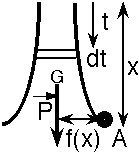## Writing the equation:

Let A be a point on the edge of the Tower. Let x be the distance
between the top of the Tower and A. Let P(x) be the weight of
the part of the Tower above A, up to the top of the Tower.

Let f(x) be the half-width of the Tower at A. The moment of
the weight of the Tower relative to point A is equal to P(x)·f(x).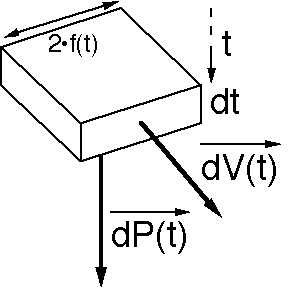Let us consider a slice of the Eiffel Tower located at a distance t
from the top of the Tower, its thickness being equal to dt.

Viewed from the top, this slice looks like a square whose
width is 2·f(t).

The forces applying on this slice are:

1. its weight dP(t), which is proportional to its volume:

2. dP(t)=k·4·f(t)2·dt
3. the horizontal wind dV(t), which is proportional to the the

4. slice's surface to the wind:
dV(t)=K·2·f(t)·dt

## Writing the differential equation:

The absolute value of the moment of dP(t) relative to point A is equal to dP(t)·f(x).
The aboslute value of the moment of dV(t) relative to point A is equal to dV(t)·(x-t).

These 2 moments relative to point A sould be equal: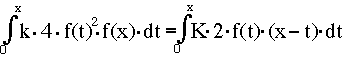Let a be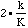. The function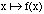, which gives the width of the Eiffel Tower as a function
of the distance from top, is a solution of the following equation: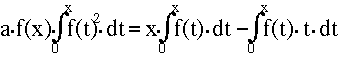### How can function f(x) be written as a combination of usual functions ?

If you can solve this equation, please E-mail me the solution: christophe@chouard.com